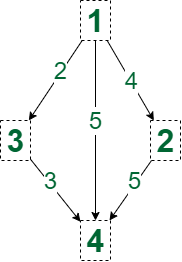Related Articles

# Number of distinct Shortest Paths from Node 1 to N in a Weighted and Directed Graph

• Difficulty Level : Medium
• Last Updated : 03 Aug, 2021

Given a directed and weighted graph of N nodes and M edges, the task is to count the number of shortest length paths between node 1 to N.

Examples:

Input: N = 4, M = 5, edges = {{1, 4, 5}, {1, 2, 4}, {2, 4, 5}, {1, 3, 2}, {3, 4, 3}}
Output: 2
Explanation: The number of shortest path from node 1 to node 4 is 2, having cost 5.Input: N = 3, M = 2, edges = {{1, 2, 4}, {1, 3, 5}}
Output: 1

Approach: The problem can be solved by the Dijkstra algorithm. Use two arrays, say dist[] to store the shortest distance from the source vertex and paths[] of size N, to store the number of different shortest paths from the source vertex to vertex N. Follow these steps below for the approach.

• Initialize a priority queue, say pq, to store the vertex number and its distance value.
• Initialize a vector of zeroes, say paths[] of size N, and make paths equals 1.
• Initialize a vector  of large numbers(1e9), say dist[] of size N, and make dist equal 0.
• Iterate while pq is not empty.
• Pop from the pq and store the vertex value in a variable, say u, and the distance value in the variable d.
• If d is greater than u, then continue.
• For every v of each vertex u, if dist[v] > dist[u]+ (edge cost of u and v), then decrease the dist[v] to dist[u] +(edge cost of u and v) and assign the number of paths of vertex u to the number of paths of vertex v.
• For every v of each vertex u, if dist[v] = dist[u] + (edge cost of u and v), then add the number of paths of vertex u to the number of paths of vertex v.
• Finally, print paths[N].

Below is the implementation of the above approach:

## C++

 `// C++ program for the above approach ` `#include ` `using` `namespace` `std; ` ` `  `const` `int` `INF = 1e9; ` `const` `int` `MAXN = 1e5 + 1; ` `vector > > g(MAXN); ` `vector<``int``> dist(MAXN); ` `vector<``int``> route(MAXN); ` ` `  `// Function to count number of shortest ` `// paths from node 1 to node N ` `void` `countDistinctShortestPaths( ` `    ``int` `n, ``int` `m, ``int` `edges[]) ` `{ ` `    ``// Storing the graph ` `    ``for` `(``int` `i = 0; i < m; ++i) { ` `        ``int` `u = edges[i], ` `            ``v = edges[i], ` `            ``c = edges[i]; ` `        ``g[u].push_back({ v, c }); ` `    ``} ` ` `  `    ``// Initializing dis array to a ` `    ``// large value ` `    ``for` `(``int` `i = 2; i <= n; ++i) { ` `        ``dist[i] = INF; ` `    ``} ` ` `  `    ``// Initialize a priority queue ` `    ``priority_queue, ` `                   ``vector >, ` `                   ``greater > > ` `        ``pq; ` `    ``pq.push({ 0, 1 }); ` ` `  `    ``// Base Cases ` `    ``dist = 0; ` `    ``route = 1; ` ` `  `    ``// Loop while priority queue is ` `    ``// not empty ` `    ``while` `(!pq.empty()) { ` `        ``int` `d = pq.top().first; ` `        ``int` `u = pq.top().second; ` `        ``pq.pop(); ` ` `  `        ``// if d is greater than distance ` `        ``// of the node ` `        ``if` `(d > dist[u]) ` `            ``continue``; ` ` `  `        ``// Traversing all its neighbours ` `        ``for` `(``auto` `e : g[u]) { ` `            ``int` `v = e.first; ` `            ``int` `c = e.second; ` `            ``if` `(c + d > dist[v]) ` `                ``continue``; ` ` `  `            ``// Path found of same distance ` `            ``if` `(c + d == dist[v]) { ` `                ``route[v] += route[u]; ` `            ``} ` ` `  `            ``// New path found for lesser ` `            ``// distance ` `            ``if` `(c + d < dist[v]) { ` `                ``dist[v] = c + d; ` `                ``route[v] = route[u]; ` ` `  `                ``// Pushing in priority ` `                ``// queue ` `                ``pq.push({ dist[v], v }); ` `            ``} ` `        ``} ` `    ``} ` `} ` ` `  `// Driver Code ` `int` `main() ` `{ ` `    ``// Given Input ` `    ``int` `n = 4; ` `    ``int` `m = 5; ` `    ``int` `edges[m] = { { 1, 4, 5 }, ` `                        ``{ 1, 2, 4 }, ` `                        ``{ 2, 4, 5 }, ` `                        ``{ 1, 3, 2 }, ` `                        ``{ 3, 4, 3 } }; ` ` `  `    ``// Function Call ` `    ``countDistinctShortestPaths(n, m, edges); ` `    ``cout << route[n] << endl; ` ` `  `    ``return` `0; ` `}`

Output:

```2
```

Time Complexity: O(MLogN)
Auxiliary Space: O(N)

Attention reader! Don’t stop learning now. Get hold of all the important DSA concepts with the DSA Self Paced Course at a student-friendly price and become industry ready.  To complete your preparation from learning a language to DS Algo and many more,  please refer Complete Interview Preparation Course.

In case you wish to attend live classes with experts, please refer DSA Live Classes for Working Professionals and Competitive Programming Live for Students.

My Personal Notes arrow_drop_up
Recommended Articles
Page :